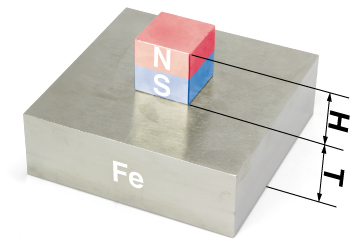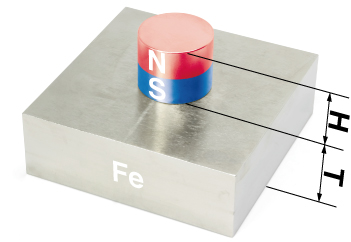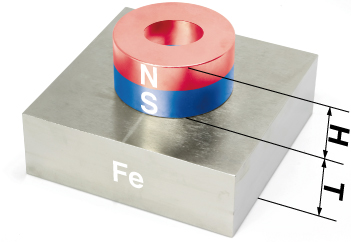## Permanent magnets

A material that continuously creates its own magnetism is called a "magnet." Artificially produced iron-based magnets contain about 1% of carbon (C) and other elements in addition to the main ingredient of iron (Fe). Since the atomic magnetism of iron is fixed in the same direction between other atoms such as carbon, a continuous magnetism toward the outside is produced and such magnets are called permanent magnets.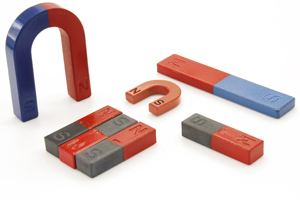## Reduced magnetization and demagnetization

The magnetization of magnets grows weaker over time but in a normal temperature environment magnetization only decreases by a very small amount over many years. Therefore, since most people think that they never lose their magnetism, such magnets are called "permanent magnets." The magnetic force of a permanent magnet depends upon the temperature environment and changes according to a temperature coefficient. When the temperature is high, the magnetic force becomes weaker, and when the temperature is low, the magnetic force becomes stronger. Permanent magnets are unable to withstand heat when continuously heated at high temperatures and the reduction in magnetism continues due to variations in the direction of the iron atoms. When a certain temperature is exceeded, the magnet is completely demagnetized. This temperature is called the Curie point or the Curie temperature after it was discovered by the French physicist Pierre Curie in 1895. The directions of the atoms can also become disordered due to vibration when a strong impact is applied to the permanent magnet which a can also lead to a reduction in magnetization.## Magnetism

The capability of a permanent magnet is often referred to as "magnetic force," but more specifically, the reactive property of a magnet is called "magnetism," the strength of the magnetism is called the "magnetic force," and the area where the magnetism operates is called the "magnetic field" or the "magnetic flux." These properties depend upon energy which is a tug-of-war between the N and S poles as the poles repel and try to move away from each other according to the two-pole property exhibited in a magnet. This magnetic energy cannot be observed visually under normal conditions. Magnetism comes out of the N pole and enters the S pole and this flow between the magnetic poles is visually represented by lines called "lines of magnetic force." This image enables the visual confirmation of magnetic energy through the use of a magnet and iron powder.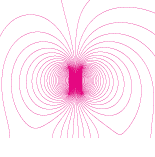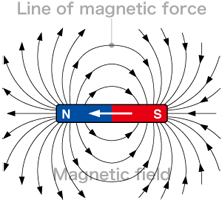## Performance evaluation of magnets

While the performance of a magnet is often abstractly referred to as "weak or strong," this performance of a magnet cannot be precisely evaluated by a third party since a sense of strength or weakness is subjective. The performance of a magnet is normally tested by conducting an evaluation based on a hysteresis curve drawn with a BH analyzer. This hysteresis curve is called a BH curve and the main indicators derived from the testing are evaluated according to international standard units such as magnetic flux density (B), coercivity (Hcb/Hcj), and maximum energy product (BH-max). Please refer to this magnetic unit converter for the magnetic units.

⇒Magnetic unit converter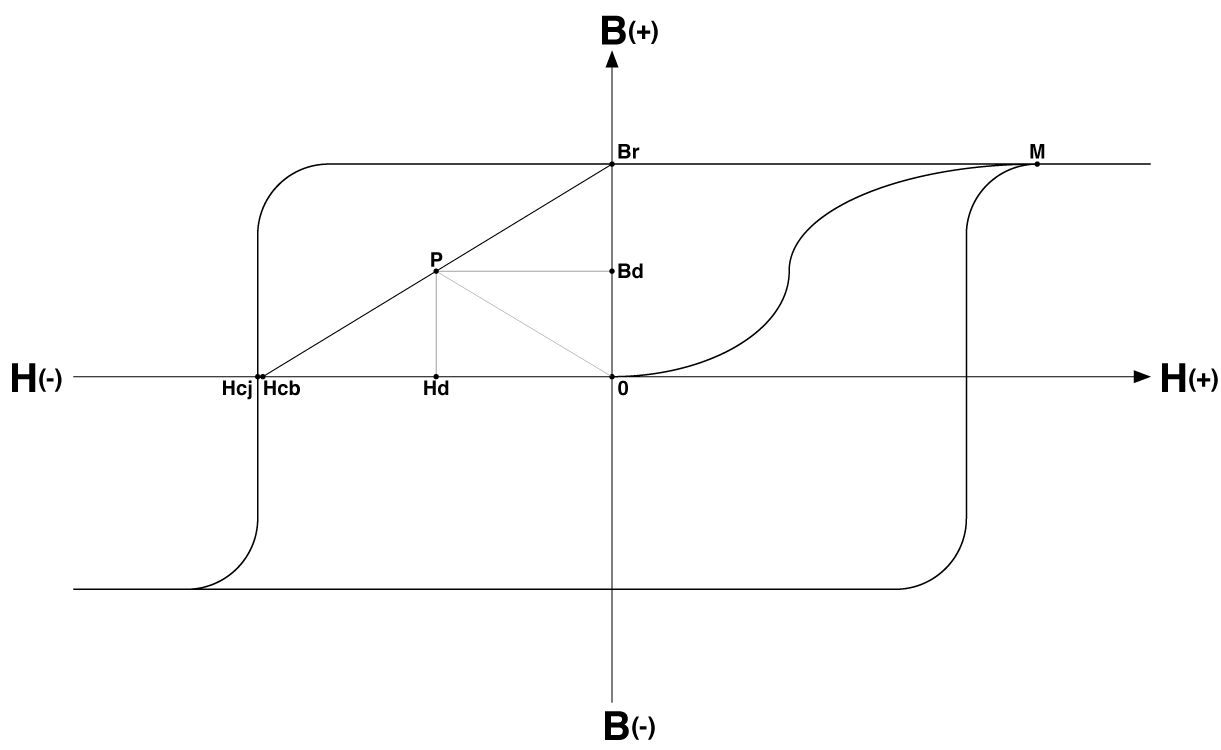## Magnetic flux density (B)

Magnetic force is exemplified by lines and multiple lines represent bundled lines of magnetic force derived from a unit surface area. Remanence (Br) indicates an amount of magnetic flux (B) held residually when a permanent magnet reaches magnetization saturation up to an M-point due to an external magnetic field (H) and then the external magnetic field (H) returns to a state of zero. The surface magnetic flux density refers to the density of the magnetic flux with respect to the outer surface of the magnet. Magnetic flux density is denoted as tesla (T) in the SI system of units (WB/m2) and as gauss (G) in the CGS system of units (Mx/cm2).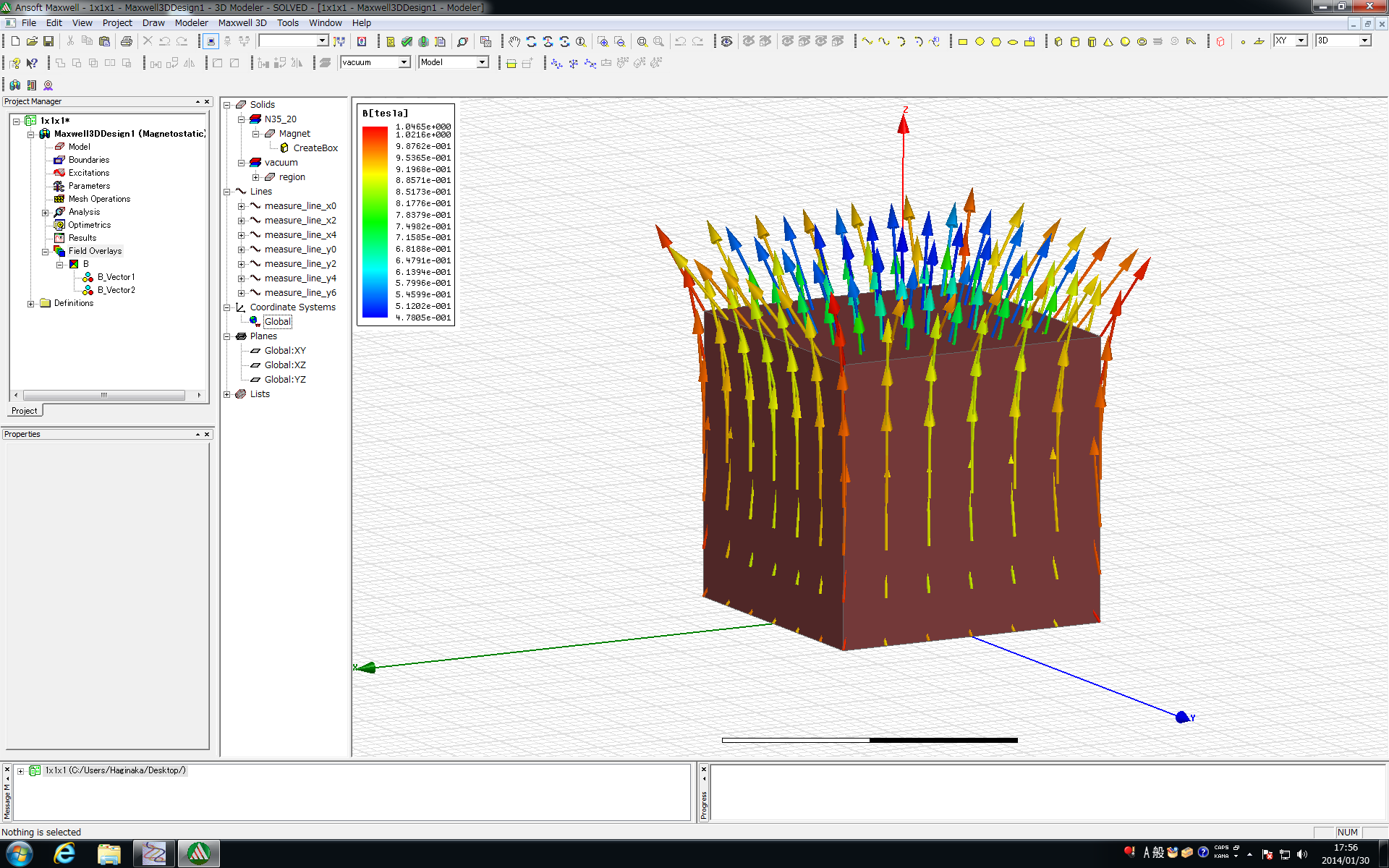## Coercivity (Hcb/Hcj)

Coercivity refers to a resistive magnetic force. Coercivity refers to the strength of an external magnetic field (H) required to return a magnetized magnetic body to a state of not being magnetized with an opposing (-) magnetic field (H). As this numerical value grows higher, resistance to a load is developed and it becomes more difficult to reduce magnetization. Coercivity is denoted by amperes per meter (A/m) in the SI system of units and denoted by oersteds (Oe) in the CGS system of units.

## Maximum energy product

The maximum value of the energy product of the magnetic field (H) and the magnetic flux (B), i.e., (Bd) * (Hd), is referred to as the maximum energy product (BH-max). The maximum energy product indicates a yardstick for the maximum magnetic flux amount which is obtained on a unit volume basis of the magnet. As this value grows higher, the straight line between the point P and the origin (0) becomes closer to 45 degrees and thus the magnet demonstrates a good balance between magnetic flux density (B) and coercivity (Hcb/Hcj). Maximum energy product is denoted by kilojoules per cubic meter (kJ/m3) in the SI system of units and by Megaoersteds (MOe) in the CGS system on units.

Adsorption force, which is also called attraction, defines the force working between two objects such as a magnet and a magnetic body containing iron. Newtons (N) are used as the representative unit for adsorption force. Basic units of mass such as kilogram-force (kgf) and pound-force (lbf) may also be used.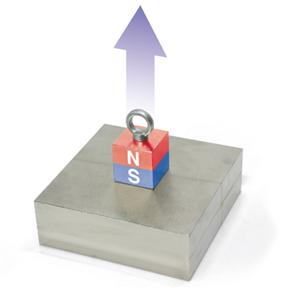Load is defined as a force produced where two points are in contact such as between a magnet and a steel plate. This the load from this force is changed depending upon friction, the state of the surface, and an impact. A slip load which indicates whether a magnet and a steel plate and the like can stay in place without slipping while withstanding a load applied horizontally, is also denoted in units of Newtons (N).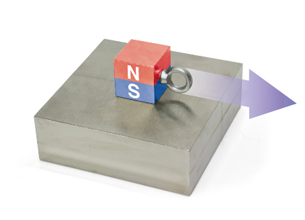The resulting numerical values tend to vary according to the usage environment and the measurement method. As a result, it is necessary to define the usage environment and the measurement method when using adsorption force in the specification of a magnet. At Magfine, these forces are defined when measured under the following measurement method and usage conditions.

## Measurement method

Adsorption force is the force when a magnet is pulled away from a steel plate perpendicular to the vertical axis and the magnet separates from the steel plate.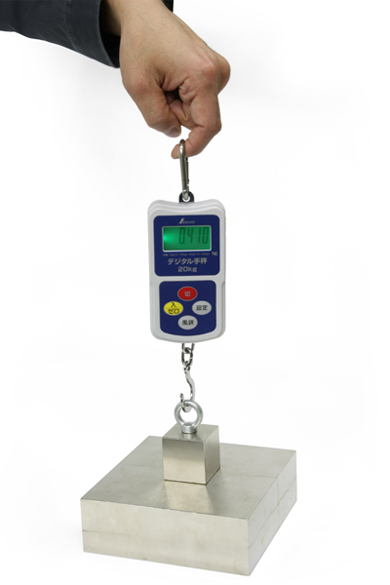A slip load is the force when a magnet is pulled parallel to the horizontal axis and the magnet moves away from the steel plate.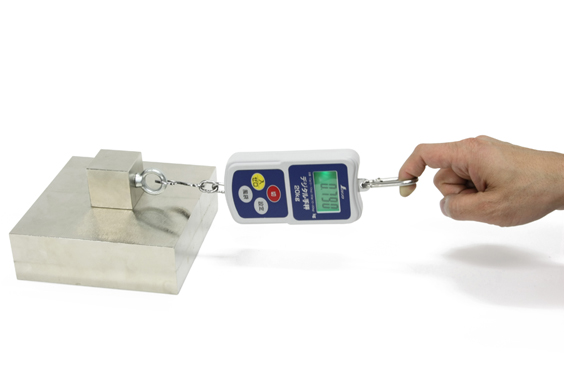## Environment conditions

1. 1. The thickness (T) of the steel plate and the thickness of the magnet (H) are as stated above.
2. 2. The magnet is placed in the center of the steel plate.
3. 3. The surface area of the steel plate is at least three times greater (300%) than the surface area of the magnet.
4. 4. The material of the steel plate is pure iron (Fe).
5. 5. The surface of the steel plate is flat without unevenness and the coefficient of friction is not considered.
6. 6. Any gap between the steel plate and the magnet is closed so that there is no gap.